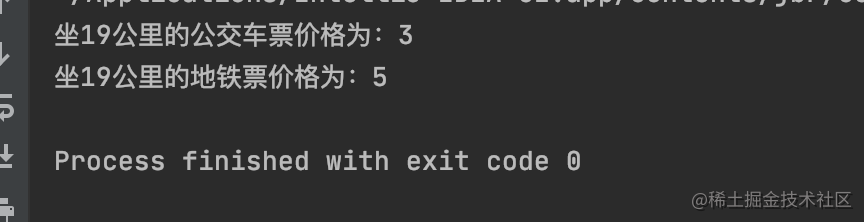# 「设计模式」策略模式

#### 使用场景

• 针对同一类型的多种处理方式,仅仅是具体行为有差别的
• 需要安全的分装多种同一类型的操作时
• 出现同一抽象类有多个子嘞,而又需要使用if-else或者switch-case来选择具体子类时候

#### 代码实现

``````
public class PriceCalculator {
private static final int BUS = 1; //公交车类型
private static final int SUBWAY = 2;//地铁类型

//公交车价格计算
private static int busPrice(int km) {
int extraTotal = km - 10;  //超过10公里总距离
int extraFactor = extraTotal / 5;  //超过的距离是5公里的倍数
int fraction = extraTotal % 5; //超过的公里对5公里取余数
int price = 1 + extraFactor * 1;//价格计算

return (fraction > 0) ? ++price : price;
}

//地铁价格计算
public static int subwayPrice(int km) {
if (km <= 6) {
return 3;
} else if (km > 6 && km < 12) {
return 4;
} else if (km > 12 && km < 22) {
return 5;
} else if (km > 22 && km < 32) {
return 6;
} else {
return 7;
}

}
//计算价格
public static int calculatePrice(int km, int type) {
if (type == BUS) {
return busPrice(km);
} else if (km == SUBWAY) {
return subwayPrice(km);
}
return 0;
}

public static void main(String[] args) {
PriceCalculator priceCalculator = new PriceCalculator();
System.out.println("坐19公里的公交车票价格为：" + priceCalculator.busPrice(19));
System.out.println("坐19公里的地铁票价格为：" + priceCalculator.subwayPrice(19));
}
}``````//定义一个计算价格的抽象策略接口
public interface CalculateStrategy {
/**
* 按照距离计算价格
* @param km 公里
* @return 返回价格
*/
int calculatePrice(int km);
}

``````public class BusStrategy implements CalculateStrategy {
@Override
public int calculatePrice(int km) {  //北京公交车 10公里之内1元，超过10公里之后每增加1元可以乘坐5公里
int extraTotal = km - 10;  //超过10公里总距离
int extraFactor = extraTotal / 5;  //超过的距离是5公里的倍数
int fraction = extraTotal % 5; //超过的公里对5公里取余数
int price = 1 + extraFactor * 1;//价格计算

return (fraction > 0) ? ++price : price;
}
}

``````//地铁出行策略类
public class SubwayStrategy implements CalculateStrategy {
@Override
public int calculatePrice(int km) {
if (km <= 6) {
return 3;
} else if (km > 6 && km < 12) {
return 4;
} else if (km > 12 && km < 22) {
return 5;
} else if (km > 22 && km < 32) {
return 6;
} else {
return 7;
}
}
}

``````//TAXI 计算策略类
public class TaxiStrategy implements CalculateStrategy {
@Override
public int calculatePrice(int km) {
return km * 2;
}
}

``````//操作策略的上下文环境
public class TranficCalculator {

public static void main(String[] args) {
TranficCalculator tranficCalculator = new TranficCalculator();
tranficCalculator.setCalculateStrategy(new BusStrategy());
System.out.println("公交车19公里的价格：" + tranficCalculator.calculatePrice(19));
tranficCalculator.setCalculateStrategy(new TaxiStrategy());
System.out.println("Taxi19公里的价格：" + tranficCalculator.calculatePrice(19));
}
private CalculateStrategy calculateStrategy;

public void setCalculateStrategy(CalculateStrategy calculateStrategy) {
this.calculateStrategy = calculateStrategy;
}

public int calculatePrice(int km) {
return calculateStrategy.calculatePrice(km);
}
}

#### 总结

##### 优点
• 结构清晰明了,使用简单直观.
• 耦合度相对之前的代码来说较低,扩展方便,可以快速扩展.
• 操作封装更为彻底,数据更加安全,类职责单一.
##### 缺点
• 随着策略的增加,子类也变得多,增加类的代码量.